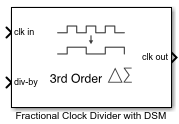Documentation

# Fractional Clock Divider with DSM

Delta Sigma Modulator based fractional clock divider

• Library:
• Mixed-Signal Blockset / PLL / Building Blocks## Description

Using delta sigma (Δ-Σ) modulation technique, a Fractional Clock Divider with DSM reduces the primary fractional spurs by spreading out the range over which the div-by value is varied. This block allows delta sigma modulation of up to 4th order.

## Ports

### Input

expand all

Input clock frequency that needs to be divided, specified as a scalar. In a phase-locked loop (PLL) system, the clk in port is connected to the output of a VCO block.

Data Types: `double`

Ratio of output to input clock frequency, specified as a fractional scalar. The value at the div-by port, N.FF, is split into two parts: the integer part (N) and the fractional part (.FF).

For an nth-order delta sigma modulator, the value at the div-by port is achieved by varying N between 2n different integer values.

### Note

For an nth order delta sigma modulator, use a value ≥ 2n at the div-by port.

Data Types: `double`

### Output

expand all

Output clock frequency, specified as a scalar. In a PLL system, the clk out port is connected to the feedback input port of a PFD block. The output at the clk out port is a square pulse train of 1 V amplitude.

Data Types: `double`

## Parameters

expand all

The order of the delta sigma modulator.

For an nth-order of the delta sigma modulator, the value at the div-by port is achieved by varying the N counter value between 2n different values. Modulator order defines the range of values by which the signal at the clk in port will be divided, providing a division effect similar to N.FF value at the div-by port.

#### Programmatic Use

• Use `get_param(gcb,'dsm')` to view the current Delta Sigma Modulator order.

• Use `set_param(gcb,'dsm',value)` to set Delta Sigma Modulator order to a specific value.

Select to enable increased buffer size during simulation. This increases the buffer size of the Logic Decision inside the Fractional Clock Divider with DSM block. By default, this option is deselected.

Number of samples of the input buffering available during simulation, specified as a positive integer scalar. This sets the buffer size of the Logic Decision inside the Fractional Clock Divider with DSM block.

Selecting different simulation solver or sampling strategies can change the number of input samples needed to produce an accurate output sample. Set the Buffer size to a large enough value so that the input buffer contains all the input samples required.

#### Dependencies

This parameter is only available when Enable increased buffer size option is selected in the Block Parameters dialog box.

#### Programmatic Use

• Use `get_param(gcb,'NBuffer')` to view the current value of Buffer size.

• Use `set_param(gcb,'NBuffer',value)` to set Buffer size to a specific value.